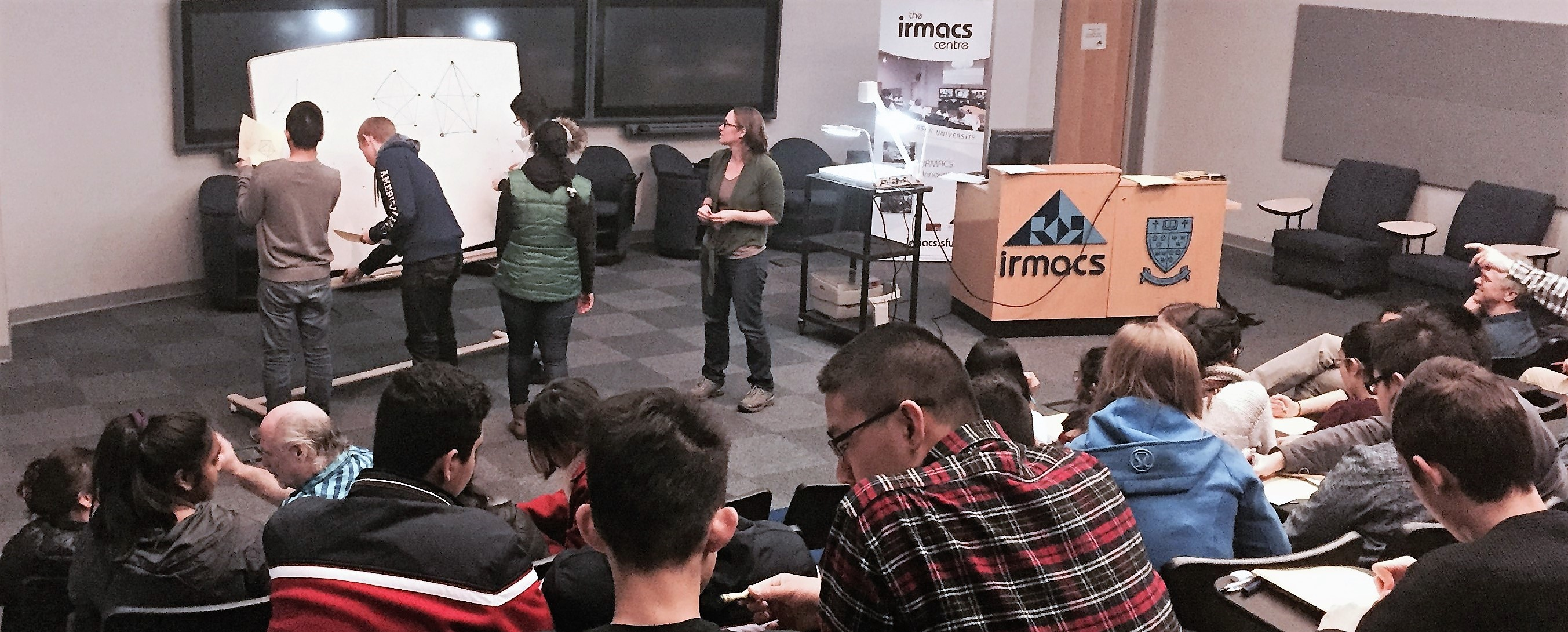### Current Students:

please see Canvas for course information.

### Sample Materials

Webcrawling activity
Appropriate for highschool students. Very little prerequisite knowledge.
A number of pages are nothing but links to each other. Students start by mapping the link structure, as a webcrawler might. Then, we examine the system as a random walk.

Graph Theory Worksheet
Appropriate for university students. Very little prerequisite knowledge.
I used this worksheet for a workshop introducing the topic of graph theory to students in the Education Justice Project at Danville Correctional Center, co-lead with Sarka Petrickova. Some of its contents are original; others are taken from various internet sources.

Geometric Sequences Worksheet
Appropriate for students who have recently been introduced to geometric sequences and have some basic mathematical maturity.
I used this worksheet with my Fall 2014 Preparation for Calculus Class. The general consensus was that they appreciated the use of examples to make the concepts more intuitive, but the large block of text at the end was surprisingly intimidating.

Matrix Theory Worksheet
Appropriate for students who have recently been introduced to matrix operations.
I used this worksheet in my Fall 2013 Introduction to Matrix Theory class to highlight important points about basic matrix algebra.Exercise 4 helped students get used to calculating with matrices, providing problems that were difficult to compute naively, but trivial to compute after some simplification. The final exercise is a scenario about cell-phone plans designed to demonstrate the usefulness of the standard definition of matrix multiplication, which students frequently find confusing.

Matrix Theory Problem Set
Appropriate for students in an introductory matrix theory course.
My Fall 2013 matrix theory students wanted extra problems to work on, so each week I created a problem bank and posted online the problems, as well as a separate solutions key. This is one of those keys.